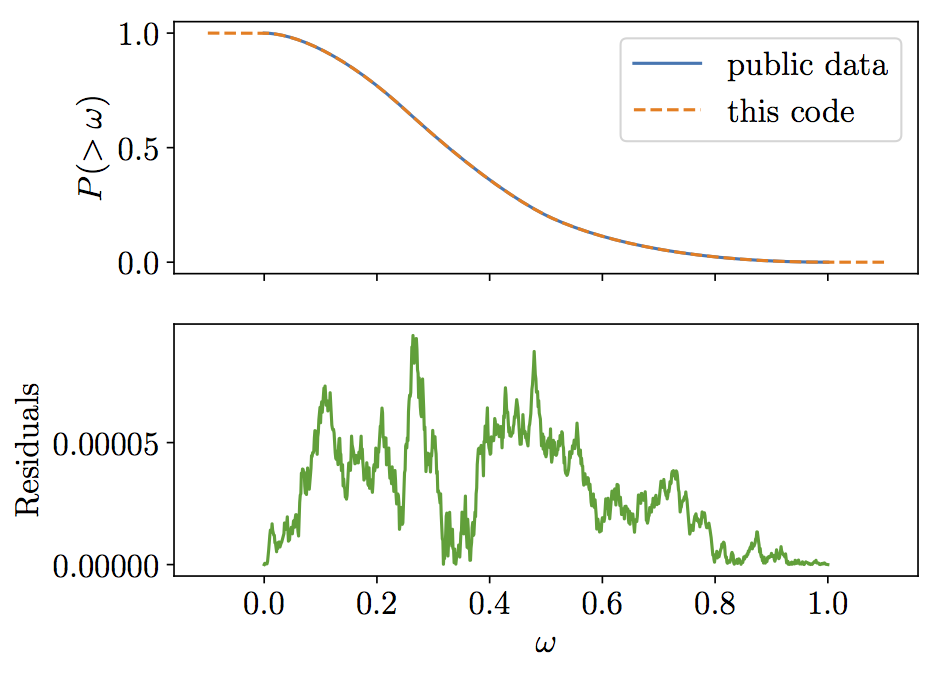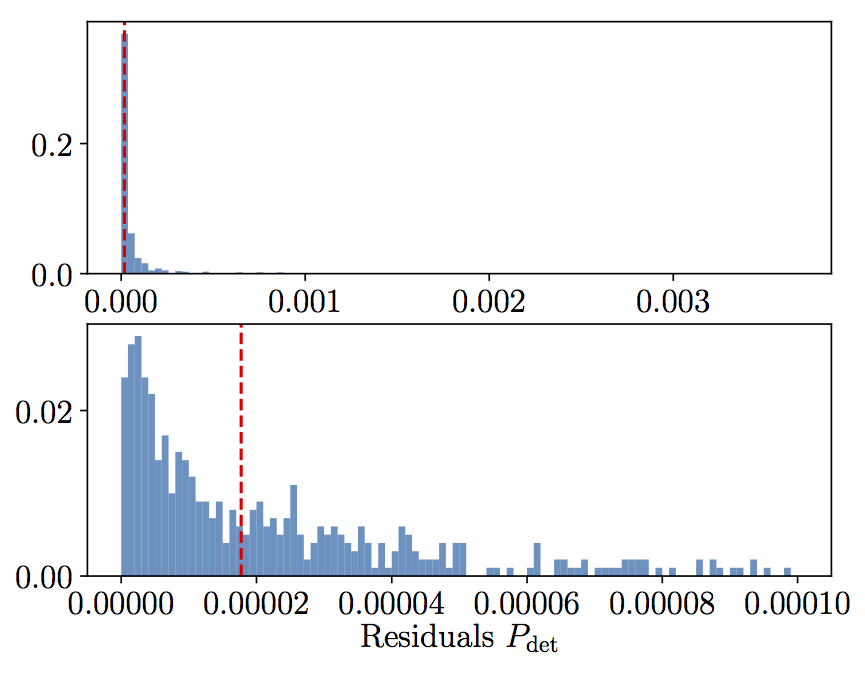# Davide Gerosa# gwdet: Detectability of gravitational-wave signals from compact binary coalescences

Check me out on github.com/dgerosa/gwdet.

This is a short python module to compute the probability of detecting a gravitational-wave signal from compact binaries averaging over sky-location and source inclination.

The detectability function is defined by Finn and Chernoff in arxiv:9301003 as a function of the projection parameter Theta (their Eq. 3.31). Here however, we follow the notation of Dominik+ in arxiv:1405.7016, which defined w= Theta/4 such that 0<=w<=1. We are interested in the cumulative distribution P(>w) of the projection parameter w (see their Eq. A.1). This gives the probabilty that an elliptically polarized gravitational-wave signal (like that of a binary) will be detected taking into account the antenna pattern of the detector and the inclination of the source (here we consider a single detector, even if in the real world we deal with networks…).

For a given (non-spinning) compact binary with masses m1 and m2 at redshift z, you first need to compute its signal-to-noise ratio snr_opt assuming optimal orientation and location (i.e. the source is face on, overhead the detector). Then specify a threshold, say 8, above which you consider the signal detectable. The probabilty that a specific binary will be detected is just P(w=8/snr_opt).

Right now this code can only handle non-spinning systems. I will generalize it to spinning sources, eventually (any help is very welcome! Send a me a pull request).

### Cite me...

If you use this software in a scientific publication, I kindly ask you to cite its DOI:This code is developed and maintained by Davide Gerosa. To report bugs, please open an issue on GitHub. If you want to contact me, it's `[email protected]`.

### Results

`gwdet` has been used in the following papers:

## Installation and checkpoints

You can install this module using pip

``````pip install gwdet
``````

Dependancies include `numpy`, `scipy`,`matplotlib`,`astropy`,`requests` and `pathos` will be installed automatically if not present. The LIGO software `lal` and `pycbc` are needed to use this code with values other than the default ones (see here for a short guide I wrote).

If you limit yourself to the default values, I provide some checkpoints files which let you use the code without installing any LIGO software. In any case, even if you have `lal`, dowloading these checkpoints will save you a lot of computational time. When you use the code with defaults parameters for the first time, a message like the following will be printed out:

``````[gwdet] You are using defaults values. You can download this interpolant. Use:
curl ....
``````

To download the checkpoints, just execute that whole command starting with `curl`. There are two checkpoint files of ~5MB and ~200MB. Note that these files will not be removed if you uninstall the module via `pip uninstall` , you will need to remove them manually. If you install `gwdet` in a virtual environment, these checkpoint files will live in the environment directory and will be removed when/if you delete the environment.

## Usage

This code has two classes only, `averageangles` and `detectability`. You first need to create an instance of each class and then use them.

The default usage is

``````p=gwdet.averageangles()
w=0.5 # Projection parameter
print(p(w)) # Fraction of detectabile sources

p=gwdet.detectability()
m1=10. # Component mass in Msun
m2=10. # Component mass in Msun
z=0.1  # Redshift
print(p(m1,m2,z))  # Fraction of detectabile sources
``````

### averageangles

Compute the detection probability, averaged over all angles (sky location, polarization, inclination, etc), as a function of the projection parameter w.

``````p = averageangles(  directory=os.path.dirname(__file__),
binfile=None,
mcn=int(1e8),
mcbins=int(1e5) )

det = p(w) # with 0<=w<=1
``````
##### Parameters:
• `directory`: where checkpoints are stored (default is the module location)
• `binfile`: checkpoint file (if `None` computed from other kwargs)
• `mcn`: resolution parameter (number of Monte Carlo samples)
• `mcbins`: resolution parameter (number of interpolated bins)
• `w`: projection parameter 0<=w<=1, see arxiv:1405.7016 (can be float or array)
##### Returns:
• `det`: GW detectability (float or array)

### detectability

Compute the detection probability of a non-spinning compact binary.

``````p = detectability(  directory=os.path.dirname(__file__),
binfile=None,
binfilepdet=None,
approximant='IMRPhenomD',
psd='aLIGOZeroDetHighPower',
psd_from_path=False,
psd_path=None,
is_asd_file=None,
flow=10.,
deltaf=1./40.,
snrthreshold=8.,
massmin=1.,
massmax=100.,
zmin=1e-4,
zmax=2.2,
mc1d=int(200),
mcn=int(1e8),
mcbins=int(1e5),
parallel=True,
screen=False)

det = p(m1,m2,z)
``````
##### Parameters:
• `directory`: where checkpoints are stored (default is the module location)
• `binfile`: checkpoint file (if None computed from other kwargs)
• `binfilepdet`: checkpoint file (if None computed from other kwargs)
• `approximant`: waveform appriximant used to compute SNRs. Available list: `pycbc.waveform.waveform.print_fd_approximants()`
• `psd`: power spectral density used to compute SNRs. Available list: `pycbc.psd.analytical.get_lalsim_psd_list()`
• `psd_from_path`: load psd using `pycbc.psd.from_txt` (default False)
• `psd_path`: power spectral density used to compute SNRs. Provide path to `.txt` file (default None)
• `is_asd_file`: check whether the `txt` file contains amplitude or power spectral density (default True)
• `flow`: starting frequency in SNR calculations
• `deltaf`: resolution parameter (frequency sampling)
• `snrthreshold`: minimum detectable signal
• `massmin`,`massax`: limits on the component masses in Msun. Interpolated inside, extrapolated outside
• `zmin`,`zmax`: limits on the redshift. Interpolated inside, extrapolated outside
• `mc1d`: resolution parameter (number of grid point per dimension)
• `mcn`: resolution parameter (number of Monte Carlo samples)
• `mcbins`: resolution parameter (number of interpolated bins)
• `parallel`: use parallel jobs on all CPUs available
• `screen`: debug option, prints all SNRs computed
• `m1`: component mass in Msun (can be float or array)
• `m2`: component mass in Msun (can be float or array)
• `z`: redshift (can be float or array)
##### Returns:
• `det`: GW detectability (float or array)

## Checks and performance

Here I first compare the performance of the P(w) interpolator implemented in `averageangles` against public data from Emanuele Berti's website. The agreement is excellent and the residuals are just numerical noise. This plot can be generated with:

``````gwdet.compare_Pw()
``````Seconly, I compare the perfomance of the P(m1,m2,z) interpolator of `detectability` against 1000 bruce force SNR computations from `lal`. Altough occasional mismathces of 3% are found, the median residuals are as small as ~1e-5. This plot can be generated with:

``````gwdet.compare_Psnr()
``````